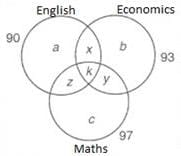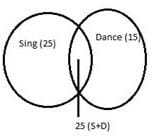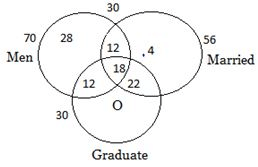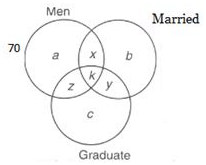UPSC  >  Questions With Answers: Venn Diagrams

# Questions With Answers: Venn Diagrams Notes | Study CSAT Preparation for UPSC CSE - UPSC

## Document Description: Questions With Answers: Venn Diagrams for UPSC 2022 is part of CSAT Preparation for UPSC CSE preparation. The notes and questions for Questions With Answers: Venn Diagrams have been prepared according to the UPSC exam syllabus. Information about Questions With Answers: Venn Diagrams covers topics like and Questions With Answers: Venn Diagrams Example, for UPSC 2022 Exam. Find important definitions, questions, notes, meanings, examples, exercises and tests below for Questions With Answers: Venn Diagrams.

Introduction of Questions With Answers: Venn Diagrams in English is available as part of our CSAT Preparation for UPSC CSE for UPSC & Questions With Answers: Venn Diagrams in Hindi for CSAT Preparation for UPSC CSE course. Download more important topics related with notes, lectures and mock test series for UPSC Exam by signing up for free. UPSC: Questions With Answers: Venn Diagrams Notes | Study CSAT Preparation for UPSC CSE - UPSC
 1 Crore+ students have signed up on EduRev. Have you?

The Venn Diagrams from the logical reasoning section would enhance your rational thinking skills. Take the practice test at jagranjosh.com to prepare for various entrances such as CAT, CMAT, MAT, XAT, IIFT, SNAP etc.
Directions (1 -4): Read the following information and answer the questions below.
In a coaching institute there are total 170 students and they studied different subject’s viz. Economics, Maths and English.
The ratio of students studying all 3 subjects to students studying at least 2 subjects is 2:9. The ratio of students studying only one subject to students studying at least 2 subjects is 8:9.
Number of students taking Maths only exceeds number of students of Economics only by 14.
Number of students studying English only exceeds number of students of Economics only by 12. Number of students studying English, Economics, and Maths is 90, 93, 97 respectively.
1. Number of students studying all three subjects is:
a) 18
b) 12
c) 20
d) None of these
Explanation:a+b+c = α, x+y+z = β, k = γ
α+β+γ= 170
α + 2β + 3γ = 90 + 93 + 97 = 280
γ:(β+γ)= 2:9
⇒ γ : β = 2 : 7
and α:(β+γ) = 8 : 9
⇒ α:β:γ = 8 : 7 : 2
∴ α = 80, β = 70 and γ = 20
⇒ a+b+c = 80, x+y+ z = 70 ...(1)
and k = 20
Again c - b =14 and a - b = 12 ...(2)
On solving eq. (1) and (2) we get a = 30, b = 18, c = 32
Again (a +x +k + z) - (a+k) = (x + z)
= 90 - (30 + 20) = 40
and (x + y + z) - (x + z) = y =70 - 40 = 30
Similarly x = 25 and z = 15

2. Number of students studying no more than one subject is:
a) 76
b) 80
c) 60
d) can’t be determined
Explanation:a+b+c = α, x+y+z = β, k = γ
α+β+γ= 170
α + 2β + 3γ = 90 + 93 + 97 = 280
γ:(β+γ)= 2:9
⇒ γ : β = 2 : 7
and α:(β+γ) = 8 : 9
⇒ α:β:γ = 8 : 7 : 2
∴ α = 80, β = 70 and γ = 20
⇒ a+b+c = 80, x+y+ z = 70 ...(1)
and k = 20
Again c - b =14 and a - b = 12 ...(2)
On solving eq. (1) and (2) we get a = 30, b = 18, c = 32
Again (a +x +k + z) - (a+k) = (x + z)
= 90 - (30 + 20) = 40
and (x + y + z) - (x + z) = y =70 - 40 = 30
Similarly x = 25 and z = 15

3. Number of students using exactly two subjects is:
a) 38
b) 55
c) 70
d) none of these
Explanation:a+b+c = α, x+y+z = β, k = γ
α+β+γ= 170
α + 2β + 3γ = 90 + 93 + 97 = 280
γ:(β+γ)= 2:9
⇒ γ : β = 2 : 7
and α:(β+γ) = 8 : 9
⇒ α:β:γ = 8 : 7 : 2
∴ α = 80, β = 70 and γ = 20
⇒ a+b+c = 80, x+y+ z = 70 ...(1)
and k = 20
Again c - b =14 and a - b = 12 ...(2)
On solving eq. (1) and (2) we get a = 30, b = 18, c = 32
Again (a +x +k + z) - (a+k) = (x + z)
= 90 - (30 + 20) = 40
and (x + y + z) - (x + z) = y =70 - 40 = 30
Similarly x = 25 and z = 15

4. The number of students who are studying both Economics and Maths but not English is:
a) 23
b) 40
c) 36
d) data insufficient
Explanation:a+b+c = α, x+y+z = β, k = γ
α+β+γ= 170
α + 2β + 3γ = 90 + 93 + 97 = 280
γ:(β+γ)= 2:9
⇒ γ : β = 2 : 7
and α:(β+γ) = 8 : 9
⇒ α:β:γ = 8 : 7 : 2
∴ α = 80, β = 70 and γ = 20
⇒ a+b+c = 80, x+y+ z = 70 ...(1)
and k = 20
Again c - b =14 and a - b = 12 ...(2)
On solving eq. (1) and (2) we get a = 30, b = 18, c = 32
Again (a +x +k + z) - (a+k) = (x + z)
= 90 - (30 + 20) = 40
and (x + y + z) - (x + z) = y =70 - 40 = 30
Similarly x = 25 and z = 15

Directions (5 - 7): Read the following information and answer the questions below.
50 students sing, 60 students do not dance and 25 students do both in a class of 100 students.
5. How many students neither sing nor dance?
a) 65
b) 35
c) 15
d) Cannot be determined
Explanation: Students who neither sing nor dance = Total students – Students who do sing alone, dance alone and do both
From Venn diagram, Students who neither sing nor dance = 100 - (25+25+15) = 35
(See the Venn diagram and explanation at the end of the questions)Given,
Total students = 100
Number of students who sing = 50
Number of students who do not dance = 60
So, number of students who dance = 100-60= 40 (Total students- Students who do not dance)
Students who dance and sing = 25 (Overlapping portion in the Venn diagram)
Number of students who sing alone = 50-25 = 25 (Students who sing- Students who sing and dance)
Number of students who dance alone= 40-25 = 15 (Students who dance- Students who sing and dance)

6. How many students only dance?
a) 10
b) 15
c) 20
d) Cannot be determined
Explanation: Students who neither sing nor dance = Total students – Students who do sing alone, dance alone and do both
From Venn diagram, Students who neither sing nor dance = 100 - (25+25+15) = 35
(See the Venn diagram and explanation at the end of the questions)Given,
Total students = 100
Number of students who sing = 50
Number of students who do not dance = 60
So, number of students who dance = 100-60= 40 (Total students- Students who do not dance)
Students who dance and sing = 25 (Overlapping portion in the Venn diagram)
Number of students who sing alone = 50-25 = 25 (Students who sing- Students who sing and dance)
Number of students who dance alone= 40-25 = 15 (Students who dance- Students who sing and dance)

7. How many students at least do either sing or dance?
a) 60
b) 50
c) 75
d) 65
Explanation: Students who neither sing nor dance = Total students – Students who do sing alone, dance alone and do both
From Venn diagram, Students who neither sing nor dance = 100 - (25+25+15) = 35
(See the Venn diagram and explanation at the end of the questions)Given,
Total students = 100
Number of students who sing = 50
Number of students who do not dance = 60
So, number of students who dance = 100-60 = 40 (Total students- Students who do not dance)
Students who dance and sing = 25 (Overlapping portion in the Venn diagram)
Number of students who sing alone = 50-25 = 25 (Students who sing- Students who sing and dance)
Number of students who dance alone = 40-25 = 15 (Students who dance- Students who sing and dance)

Direction (8-10): Read the following information and answer the questions below.
There are 120 employees who work for Airtel Pvt. Ltd. Mumbai, out of which 50 are women. Also:
I. 56 workers are married
II. 52 workers are graduate
III. 40 married workers are graduate of which 18 are men
IV. 30 men are graduate
V. 30 men are married.
8. How many unmarried women are graduate?
a) 22
b) 16
c) 0
d) can’t be determined
Explanation: No one unmarried woman is graduate.Total number of employees = 120
Women = 50
Men = 70
Married workers = 56
Graduate workers = 52
a → unmarried men who are not graduate
b → married women who are not graduate
c → unmarried women who are graduate
x → married men who are not graduate
y → married women who are graduate
z → unmarried men who are graduate
k → married men who are graduate
p → unmarried women who are not graduate
According to the given information the Venn diagram can be completed as given below.

9. How many unmarried women are graduate?
a) 21
b) 24
c) 19
d) none of these
Explanation: Number of unmarried women = 120 - [28 + 4 + 12 + 12 + 22 + 18] = 24Total number of employees = 120
Women = 50
Men = 70
Married workers = 56
Graduate workers = 52
a → unmarried men who are not graduate
b → married women who are not graduate
c → unmarried women who are graduate
x → married men who are not graduate
y → married women who are graduate
z → unmarried men who are graduate
k → married men who are graduate
p → unmarried women who are not graduate
According to the given information the Venn diagram can be completed as given below.

10. How many graduate men are married?
a) 18
b) 15
c) 13
d) none of these
Explanation: There are 18 graduate men who are married.Total number of employees = 120
Women = 50
Men = 70
Married workers = 56
Graduate workers = 52
a → unmarried men who are not graduate
b → married women who are not graduate
c → unmarried women who are graduate
x → married men who are not graduate
y → married women who are graduate
z → unmarried men who are graduate
k → married men who are graduate
p → unmarried women who are not graduate
According to the given information the Venn diagram can be completed as given below.

The document Questions With Answers: Venn Diagrams Notes | Study CSAT Preparation for UPSC CSE - UPSC is a part of the UPSC Course CSAT Preparation for UPSC CSE.
All you need of UPSC at this link: UPSC

## CSAT Preparation for UPSC CSE

72 videos|64 docs|92 tests
 Use Code STAYHOME200 and get INR 200 additional OFF

## CSAT Preparation for UPSC CSE

72 videos|64 docs|92 tests

### How to Prepare for UPSC

Read our guide to prepare for UPSC which is created by Toppers & the best Teachers

Track your progress, build streaks, highlight & save important lessons and more!

,

,

,

,

,

,

,

,

,

,

,

,

,

,

,

,

,

,

,

,

,

;